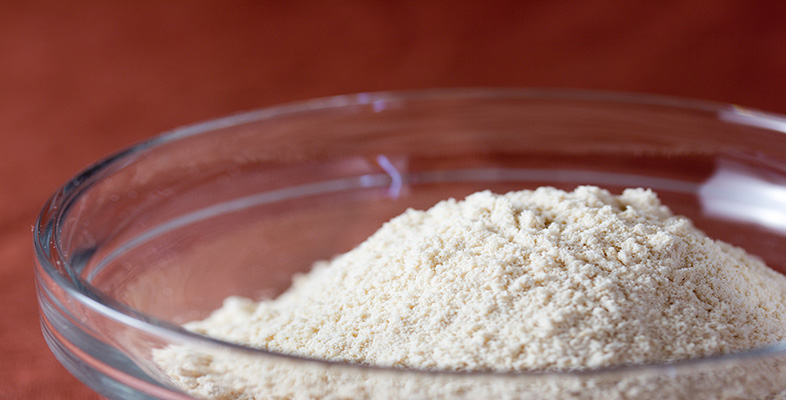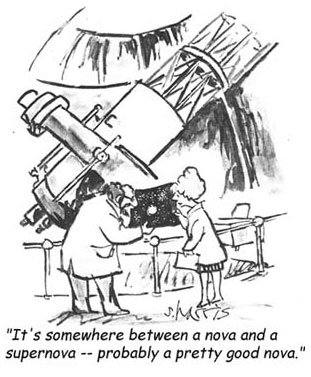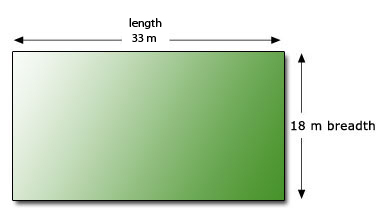Rounding and estimation

This free course is available to start right now. Review the full course description and key learning outcomes and create an account and enrol if you want a free statement of participation.

Free course

2 Estimation

2.1 Using estimationsApproximations are most useful when it comes to making rough estimates – like adding up a bill quickly to see if it is about right or checking a calculation. When using a calculator it is very important that you have an independent means (by estimation) of judging whether your answer is correct, or at least plausible. To make a rough estimate the numbers must be easy to work with, so being able to round numbers is an extremely useful skill.

For different purposes different degrees of approximation may be appropriate. Be careful about the level of accuracy of an estimate.

Example 5

Dave and Sally are planning to travel from Newcastle to London, then to Gloucester, then to Liverpool and finally home to Newcastle.

• Newcastle to London 282

• London to Gloucester 104

• Gloucester to Liverpool 146

• Liverpool to Newcastle 170

• (a) Make a rough estimate of the total distance travelled.

• (b) Petrol is estimated at £1 a litre. Their car does about 8 miles to the litre. Estimate their total petrol costs.

• (a) Get a rough estimate by rounding to the nearest 10.

• Newcastle − London is about 280 miles.

• London − Gloucester is about 100 miles.

• Gloucester − Liverpool is about 150 miles.

• Liverpool − Newcastle is about 170 miles.

So the total distance is about 700 miles. The signmeans ‘is approximately equal to’, so this can be written as

total distance700 miles.

(The same estimate, 700 miles, is also attained by rounding each figure to the nearest 100 in the first place! There are a number of ways of making rough estimates.)

• (b) To estimate the petrol costs, take the fuel consumption to be 10 miles per litre (as it is easier to divide by 10 than by 8). This gives the number of litres needed as about 700/10 = 70 litres. At £1 per litre the cost is about £70.

Since some information is given only to one or two significant figures, the estimate cannot be more accurate.

Example 6

A rectangular lawn measures 33 m by 18 m. Make a rough estimate of its area. The lawn fertilizer to be used on it is suitable (when diluted) for about 100 square metres for each packet of fertilizer. How many packets are needed?You get a rough estimate by rounding to the nearest 10:

33 rounds down to 30;

18 rounds up to 20.

So

area30 m × 20 m600 square metres.

One packet of fertilizer covers about 100 square metres. So six packets will probably be needed.

(Area of a rectangle is length × breadth. Area is measured in square metres, square feet, etc.)

These examples illustrate that a rough estimate can give a reasonable idea of the result.

An important use of estimation is as a check for calculator work.

Example 7

Estimate 563.3 × (6.891 + 44.34) as a check on the result you get by using your calculator to do the calculation.

This is a calculation you would be unlikely to undertake by hand. But, before you reach for your calculator, you should estimate your answer.

Round to the nearest whole number (evaluate the brackets first):

6.891 + 44.347 + 4450

563.3600.

So the estimate of the calculation is

600 × 50 = 30 000.

So a rough estimate for the answer is 30 000.

You can use your calculator to find the answer exactly (using brackets). It comes to 28 858.4223, close to your estimate of 30 000.

lf you got a very different answer, you would be suspicious and should look for an error.

MU120_4M2